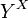# Topology of pointwise convergence

Suppose$X$ and$Y$ are topological spaces. Let$C(X,Y)$ denote the space of continuous maps from$X$ to$Y$. The topology of pointwise convergence on$C(X,Y)$ is defined in the following equivalent ways:
2. It is the topology on$C(X,Y)$ arising as the subspace topology from the product topology on the space of all functions$Y^X$.
In particular, the topology of pointwise convergence is little influenced by the topology of$X$, although the underlying set of the topology is influenced by$X$.